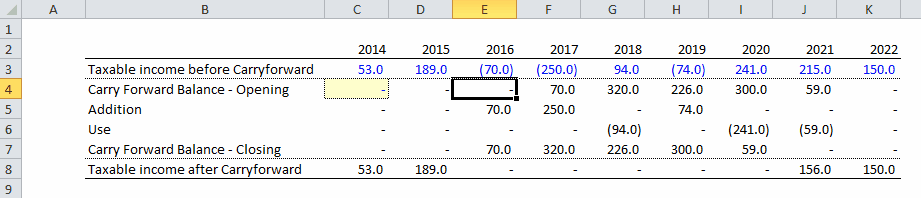top of page

## Coding

Loss Carryforward

A tax loss carryforward occurs when the taxable income of a company is negative, in this case the company is allow to carry forward the losses to use them later on when it makes profits again (depends on the tax code of each country).

The idea is to create an account that will record the tax losses when they occur and carry them forward until they can be used. For each time period there will an opening balance for that account as well as a closing balance.

Excel modeling

1. Calculate the taxable income

2. Create a table below (or wherever you want) with 4 rows: opening balance of losses carried forward, addition to balance, use of carried forward losses and closing balance of losses carried forward

3. The addition to the carryforward balance is minus the minimum between zero and the taxable income, this way if the taxable income is positive, then the formula will return 0

4. The use of carried forward losses occurs when current profits can be offset by past losses, the maximum amount of carried forward losses that can be used is the minimum between the current profits and the opening balance of carried forward losses. The reduction in carried forward losses is therefore minus the maximum between 0 and the value calculated earlier (minimum of profits and opening balance)

5. Calculate the closing balance of carried forward lossesbottom of page